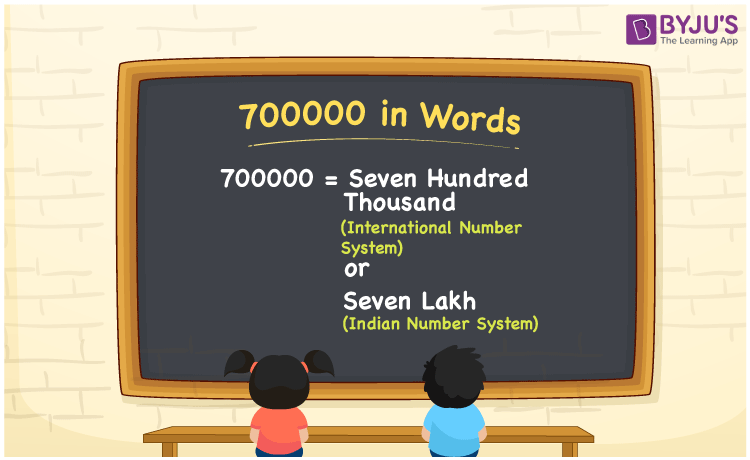# 700000 in words

700000 in words is written as Seven Lakh in the Indian Numeral System and Seven Hundred Thousand in the International Numeral system. The article on Numeral System can give you an idea about the difference between both systems. The number 700000 is used in expressions that relate to huge quantities, like money, population, Social media views, distance between celestial bodies. If expressed in terms of Money, it would be “My new job offered me Seven Lakh Rupees per annum as the package. i.e Rs 700000 per annum” Another example, “Seven Hundred Thousand dollars is a huge amount of money to have as a bank balance. It Is \$700000.”

 Numeral System → Indian Numeral System International Numeral System 700000 in words Seven Lakh Seven Hundred Thousand Seven Lakh in Numbers 7,00,000 – Seven Hundred Thousand in Numbers – 700,000

## 700000 in English Words## How to Write 700000 in Words?

We can convert 700000 to words using a place value chart. The number 700000 has 6 digits, so let’s make a chart that shows the place value up to 6 digits. Different tables to show both Numeral Systems are given.

Indian System

 Lakhs Ten Thousands Thousands Hundreds Tens Ones 7 0 0 0 0 0

Thus, we can write the expanded form as:

7 × Lakhs + 0 × Ten thousand + 0 × Thousand + 0 × Hundred + 0 × Ten + 0 × One

= 7 × 100000 + 0 × 10000 + 0 × 1000 + 0 × 100 + 0 × 10 + 0 × 1

= 700000

= Seven Lakh

International System

 Hundred Thousands Ten Thousands Thousands Hundreds Tens Ones 7 0 0 0 0 0

Thus, we can write the expanded form as:

7 × Hundred Thousand + 0 × Ten thousand + 0 × Thousand + 0 × Hundred + 0 × Ten + 0 × One

= 7 × 100000 + 0 × 10000 + 0 × 1000 + 0 × 100 + 0 × 10 + 0 × 1

= 700000

= Seven Hundred Thousand.

700000 is the natural number that is succeeded by 699999 and preceded by 700001.

700000 in words – Seven Lakh (Indian System) and Seven Hundred Thousand (International System).

Is 700000 an odd number? – No.

Is 700000 an even number? – Yes.

Is 700000 a perfect square number? – No.

Is 700000 a perfect cube number? – No.

Is 700000 a prime number? – No.

Is 700000 a composite number? – Yes.

## Solved Example

1. Write the number 700000 in expanded form.

Solution: = 7 × 100000 + 0 × 10000 + 0 × 1000 + 0 × 100 + 0 × 10 + 0 × 1

We can write 700000 = 700000 + 0 + 0 + 0 + 0 + 0

Therefore it will be

= 7 × 100000 + 0 × 10000 + 0 × 1000 + 0 × 100 + 0 × 10 + 0 × 1

## Frequently Asked Questions on 700000 in words

Q1

### How to write the number 700000 in words?

The number 700000 in words is written as Seven Lakh in the Indian System and Seven Hundred Thousand in the International System.
Q2

### 700000 is a perfect square number. True or False?

False. 700000 is not a perfect square number.
Q3

### Is 700000 divisible by 10?

Yes. 700000 is divisible by 10.